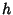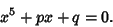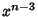## Tschirnhausen Transformation

A transformation of a Polynomial equationwhich is of the formwhereandare Polynomials anddoes not vanish at a root of. The Cubic Equation is a special case of such a transformation. Tschirnhaus (1683) showed that a Polynomial of degreecan be reduced to a form in which theandterms have 0 Coefficients. In 1786, E. S. Bring showed that a general Quintic Equation can be reduced to the formIn 1834, G. B. Jerrard showed that a Tschirnhaus transformation can be used to eliminate the,, andterms for a general Polynomial equation of degree.